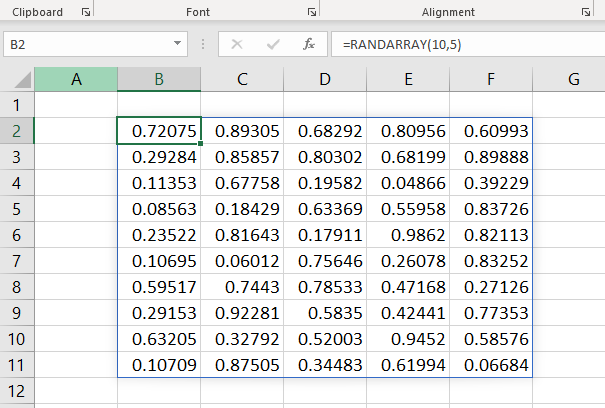# RANDARRAY FunctionThe Excel RANDARRAY function is a DYNAMIC ARRAY FUNCTION that returns an array of random numbers between the two numbers specified by us. The function also has options to define the size of the array and to determine whether the values should be decimals or whole numbers.

### Function Type

Math and trigonometry

### Purpose

Generate an array of random numbers

### Return value

An array of random numbers

### Syntax

`=RANDARRAY([rows],[columns],[min],[max],[integer]) `

### Arguments

rows – [optional] Number of rows of Array. Default value is 1.

columns – [optional] Number of of Array. Default value is 1.

min – [optional] Minimum value to return. Default value is 0.

max – [optional] Maximum value to return. Default value is 1.

integer – [optional] TRUE for integer, FALSE for decimal

### Examples

#### Example 1

Use the following formula to create an array of size 10 x 5 containing random numbers

`=RANDARRAY(10,5)`

#### Example 2

To define the limits of maximum and minimum values, use the third and fourth arguments (min & max) in the RANDARRAY function.

To generate an array of size 6 x 7 containing random numbers between 10 and 1000, use the following formula

`=RANDARRAY(6,7,10,1000)`

#### Example 3

The fifth argument of the RANDARRAY function, integer determines whether to return Integers or numbers with decimals. Use TRUE in this place to return an array of random integers.

` =RANDARRAY(6,7,10,1000,TRUE)`

The above will return an array of size 6 x 7 containing random integers between 10 and 1000.

#### Example 4

Negative numbers can also be generated using the RANDARRAY function.

The following formula will return an array of size 8 x 6 containing random integers between -512 and 789.

`=RANDARRAY(8,6,-512,789,TRUE)`